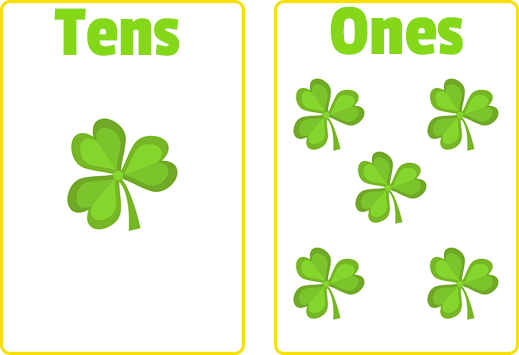# Tens and Ones

Tags: Kindergarten, CCSS.MATH.CONTENT.K.NBT.A.1, Place Value

Question 1 (Short Answer, 10 points)

What number is in the ones place?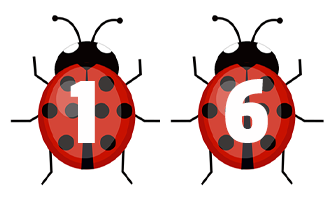Question 2 (Short Answer, 10 points)

Which number is in the tens place?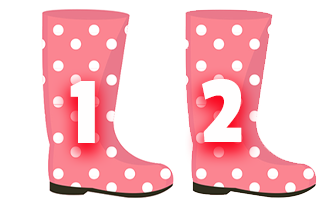Question 3 (Short Answer, 10 points)

What number is in the ones place?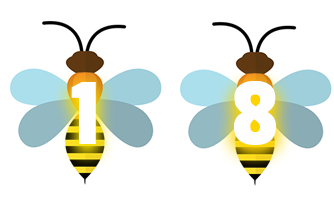Question 4 (Multiple Choice, 10 points)

How would you write this number?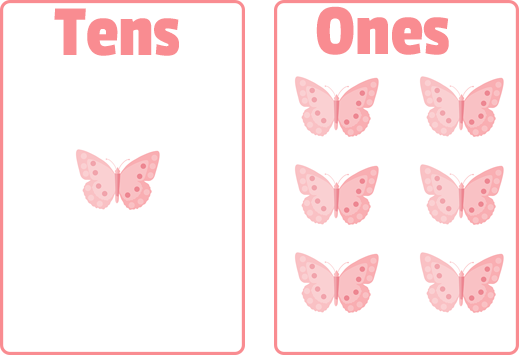Question 5 (Multiple Choice, 10 points)

How would you write this number?